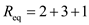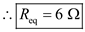Problem

# By combining the resistors in Fig. 2.36, find Req.Answer:6Ω.

By combining the resistors in Fig. 2.36, find Req.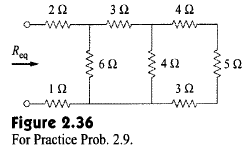#### Step-by-Step Solution

Solution 1

The given circuit is: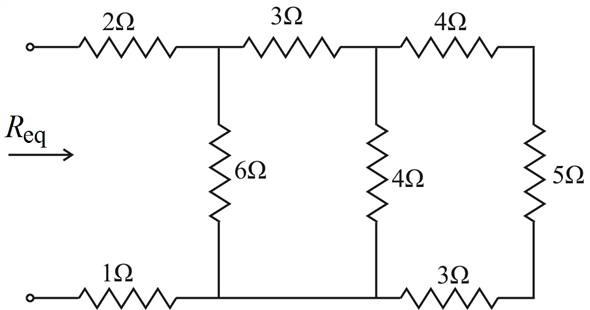To get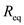, we combine resistors in series and in parallel.

The resistors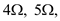and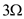are in series, so their equivalent resistance is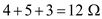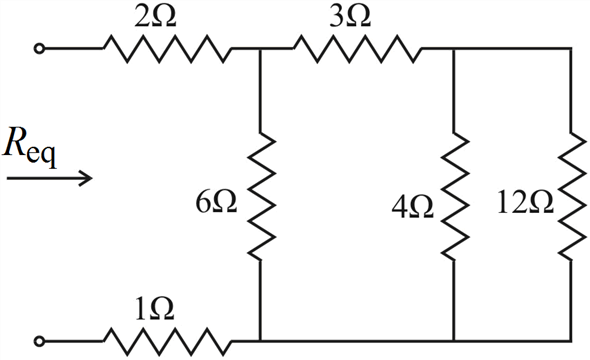The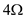and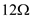resistors are in parallel, so their equivalent resistance is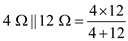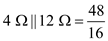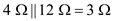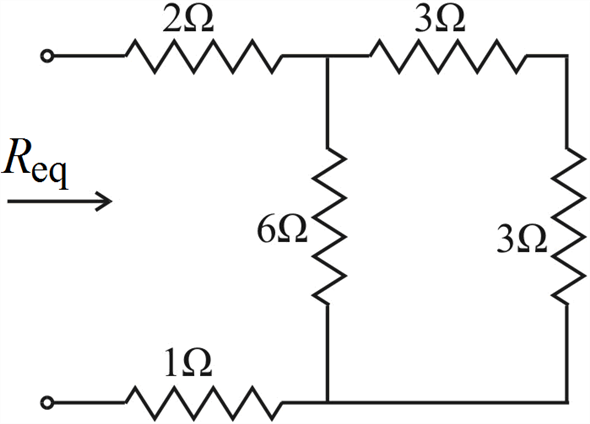Theandresistors are in series, so their equivalent resistance is,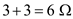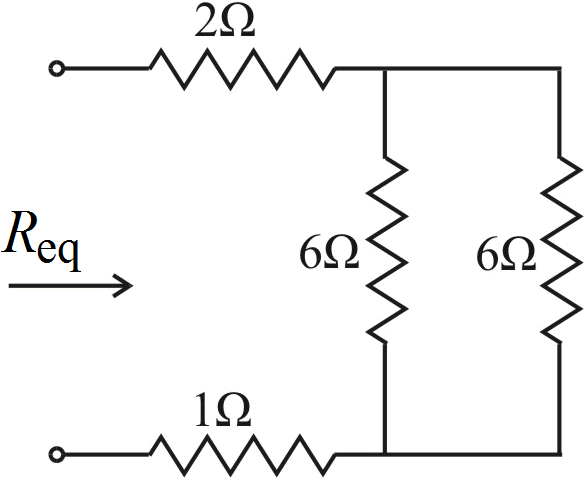The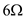andresistors are in parallel, so their equivalent resistance is,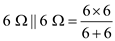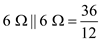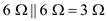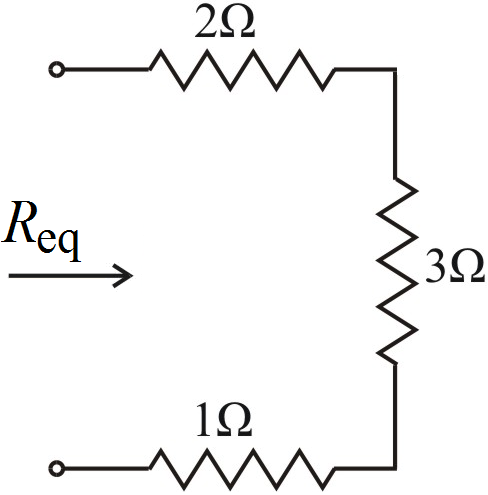From the above circuit the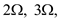and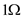resistors are in series. So their equivalent resistance is,